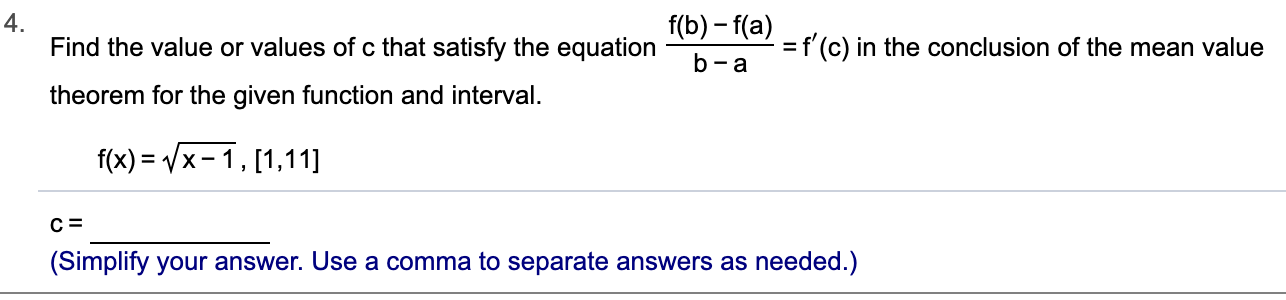# 4.Find the value or values of c that satisfy the equationf(b)-f(a)f (c) in the conclusion of the mean value- atheorem for the given function and intervalf(x) x-1, [1,11](Simplify your answer. Use a comma to separate answers as needed.)

Question
1 views

Can you help me step by step?help_outlineImage Transcriptionclose4. Find the value or values of c that satisfy the equation f(b)-f(a) f (c) in the conclusion of the mean value - a theorem for the given function and interval f(x) x-1, [1,11] (Simplify your answer. Use a comma to separate answers as needed.) fullscreen
check_circle

Step 1

Given function and interval is

Step 2

Differentiate the function and put x = c

Step 3

Now evalua...

### Want to see the full answer?

See Solution

#### Want to see this answer and more?

Solutions are written by subject experts who are available 24/7. Questions are typically answered within 1 hour.*

See Solution
*Response times may vary by subject and question.
Tagged in

### Other